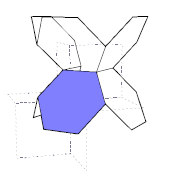Acronym shexat Name hyperbolic order 4 hexagonal tiling `©` Circumradius 1/sqrt(-2) = 0.707107 i Vertex figure  Dual x4o6o Confer uniform relatives: x3xØx∞xØ*a3*c   o4x∞o   combinatorical relatives: x5o4o   {8}-quartered x8o4o   general polytopal classes: regular   noble polytopes ExternallinksHaving the same curvature (resp. circumradius) as o4x∞o, both having even numbered polygons only and both having tetravalent vertex figures with inversional symmetry, these allow for resp. alternating layers, i.e. an corresponding laminat. That one then would have the vertex configuration [4,6,6,∞] and could be described by the generalized Dynkin diagram x3xØx∞xØ*a3*c.

Note that o4o6x further allows for a consistent halving of hexagons (connecting opposite edge midpoints), such as to derive a combinatorical variant of x5o4o. While for that one the edge lengths of the regular pentagons a-a-a-a-a- can be calculated as given below for comparison, in that decomposition the edge lengths of these non-regular pentagons a-a-b-c-b- would calculate (using the formulas provided here) as:

```x6o4o (halving each hexagon gives a topological variant of x5o4o):
P0 o-+ P1
P1 /  |
P0 o   | P2
\  |
o-+ P1
edges a: hypdist(P0,P0) = 2 arcosh[cos(π/6)/sin(π/4)] = 1.316958
edges b: hypdist(P0,P1) =   arcosh[cos(π/6)/sin(π/4)] = 0.658479
edges c: hypdist(P1,P1) = 2 arcosh[cos(π/4)/sin(π/6)] = 1.762747
deformed pentagons a-a-b-c-b-

(to be conpared to:
x5o4o
all edges alike: hypdist(P0,P0) = 2 arcosh[cos(π/5)/sin(π/4)] = 1.061275
regular pentagons a-a-a-a-a- )
```
`©©`

There exists also a series of regular modwraps of this tiling, obtained by identifying every k-th vertex on each hole (q.e. straight edge sequence here). Then it allows a representation as (possibly) infinite regular skew polyhedron, which happens to be the facial subset of hexagons of the spherical decachoron (k=3: finite modwrap), of the euclidean bitruncated cubical honeycomb (k=4), of the hyperbolic bitruncated order 5 dodecahedral tesselation (k=5), etc. This series of modwraps generally is being denoted as x6o4o|k (with according value of k).

Incidence matrix according to Dynkin symbol

```o4o6x   (N → ∞)

. . . | 3N |  4 |  4
------+----+----+---
. . x |  2 | 6N |  2
------+----+----+---
. o6x |  6 |  6 | 2N

snubbed forms: o4o6s
```

```o6x6o   (N → ∞)

. . . | 3N |  4 | 2 2
------+----+----+----
. x . |  2 | 6N | 1 1
------+----+----+----
o6x . |  6 |  6 | N *
. x6o |  6 |  6 | * N

snubbed forms: o6s6o
```